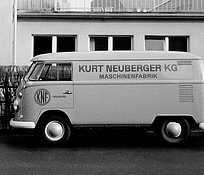General

# Mass Flow vs Volumetric Flow

The term ‘flow’ is often used without further specifications even though the distinction between mass flow and volumetric flow can make a big difference.

Imagine a gas vendor uses a volumetric flow meter to measure the amount of gas and charges by volume. Assuming the gas price per volume is constant, an observant buyer would soon realize that the same volume has a higher mass, and therefore a higher energy content, when purchased in winter. This would make buying gas in the winter cheaper. Why is that? The answer lies in the special properties of gases. When dealing with them, a distinction between ‘mass flow’ and ‘volumetric flow’ (also known as ‘volume flow’) is necessary.

## Volatile Appearance: Gases

One of the most significant differences between liquids and gases is that gases are compressible. This means the same mass of gas can vary tremendously in volume V depending on its temperature T, its pressure p and its unique properties also called specific gas constant R. This behavior is summarized by the ideal gas law, yielding:

$$pV = mRT$$.

The ideal gas equation represents the influence of pressure and temperature on the volume of a constant amount of gas mass.

## Temperature and Pressure: Important Variables

In practical terms, this means the volume of a constant amount of gas increases the hotter it gets when pressure remains constant. For example, measured at atmospheric pressure (1 bar abs.), 100 grams of dry air has a volume of ca. 78 liters at 0°C but ca. 90 liters of volume at 40°C. On the other hand, when pressure increases while the temperature is constant, the same mass of the gas occupies a smaller volume. At 1 bar abs., 100 grams of hydrogen fill a volume of 1135 liters. At 100 bar abs., the same mass of hydrogen has a volume of just ca. 11 liters.

## The Specific Gas Matters

As seen by the example above, the same mass of gas at the same temperature and pressure occupies a different volume for different gases. The reason for this is the different specific gas constant R which can vary significantly from gas to gas. For dry air the specific gas constant is $$R_{air} = 287 J/(kg K)$$ and for hydrogen it is $$R_{H_2} = 4157 J/(kg K).$$

## Mass Flow vs Volumetric Flow

With this special behavior of gases fresh in mind, it becomes clear why the term ‘flow’ has to be further specified. Flow can be quantified by either stating the mass flow rate or the volumetric flow rate. Volumetric flow is presented using units like liter per minute or cubic meter per second. Mass flow on the other hand describes the rate in which a certain mass is moved using units such as kilogram per second or gram per minute.

## Mass Flow and Volumetric Flow: Making a Difference

Demonstrated with the example from the beginning, the distinction between mass flow vs volumetric flow is not only theoretically important but can have real impact on our daily lives. Let us assume that our hypothetical gas vendor sells hydrogen in a region with strong seasonal temperature changes. This means that the gas temperature can change between 0°C in the winter and 40°C in the summer.

Therefore, 100 liters sold at the same price can range in mass between 76 grams and 88 grams. This makes it 13 % more expensive for customers to buy hydrogen in the summer. Our imaginary example illustrates how important the distinction between mass flow and volumetric flow is. It also shows why gases such as hydrogen or natural gas are mostly sold by mass not by volume.

## Which Metric to Use?

This example begs the question: which metric should be used? The answer is: it depends. For example, most calculations are a lot easier using mass flow because the mass is conserved and does not depend on temperature or pressure. However, most people do not have an intuitive understanding of gas masses. Here, volumetric flow is more tangible and accessible for practical everyday purposes.

Finally, talking about a volumetric flow, it is crucial to know the pressure, the temperature, and the kind of gas, represented by the specific gas constant of this volumetric flow. Therefore, KNF specifies in data sheets a volume flow measured at atmospheric pressure with air at room temperature. For our imaginary gas vendor from the beginning, this means that the gas should only be sold by volume if this volume is specified at a constant reference temperature and pressure. The other option would be using a mass flow meter and sell the gas by mass.

## 75 Years: KNF Celebrates Company Anniversary